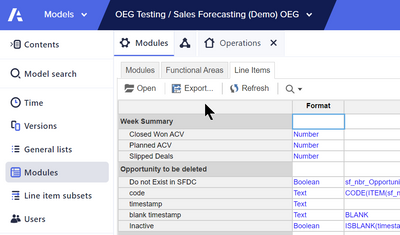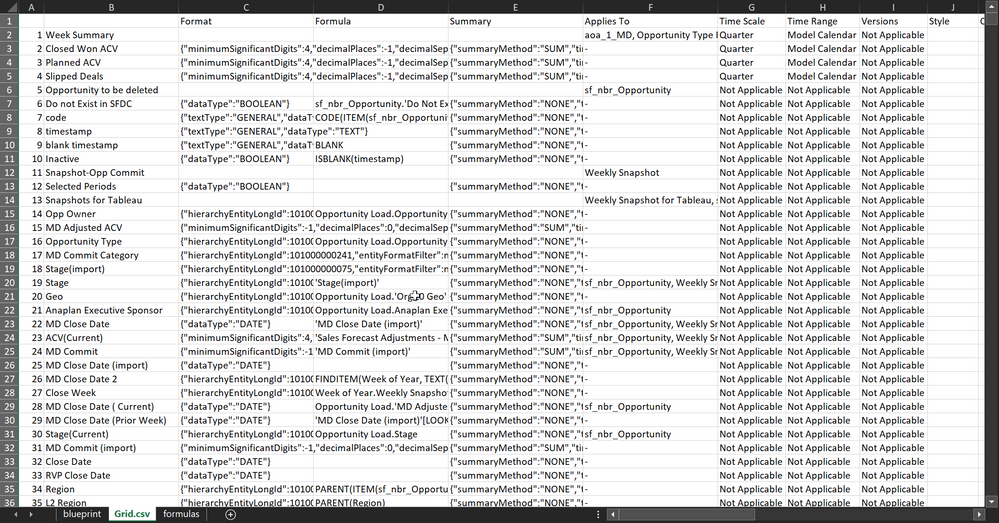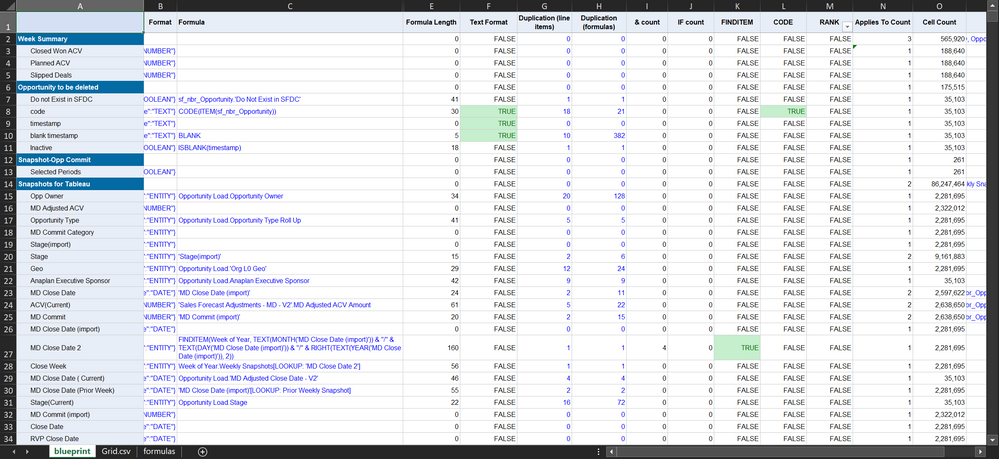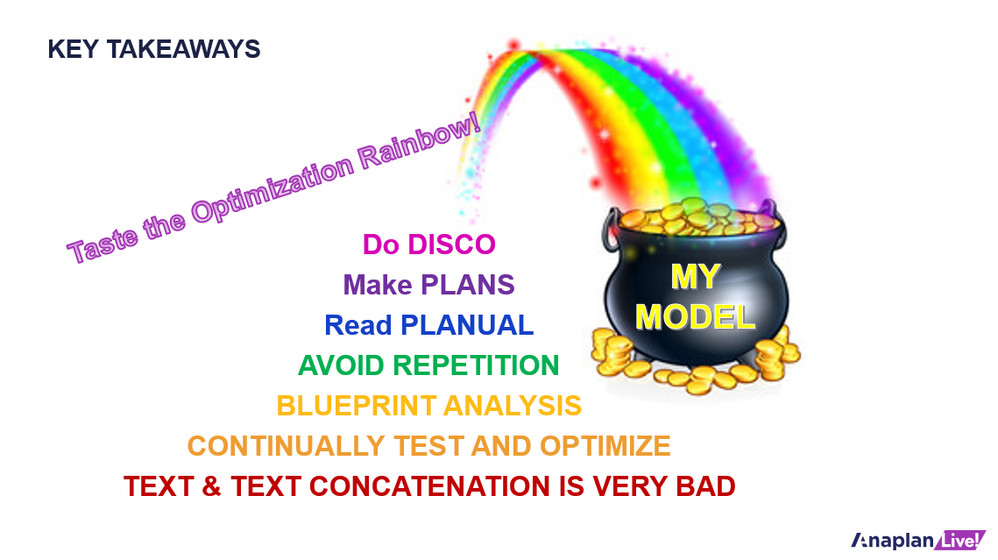# Model Optimization## Blueprint Analysis

#### What?

A model analysis technique using metadata from your Anaplan model imported in to Excel; utilizing purpose built formulas to identify line items of interest.

#### Why?

We do this so that we have some extra information to arm ourselves when trying to optimize a model.

It lets us look at every line item and apply the same rules to each and highlight line items that breach certain rules.

We can then filter the worksheet and look at which line items we consider worth investigating first; those we have highlighted as most likely to cause performance issues.

#### How?

Begin by exporting line item "blueprint" from the third tab in Modules (on the settings menu).
Export as CSV (Grid.csv)Import this in to Excel:Now we can begin to format the columns, hide those we are not interested in and add columns for analysis we want to accomplish.As an example, we've added a Formula Length column with the formula: =LEN(C2)
C2 is the formula column we have imported from the blueprint.

Here are the examples in the attached file:

 Formula Length `=LEN(C2)` Text Format `=ISNUMBER(FIND("TEXT",B2))` Duplication (line items) `=IF(C2=\$C\$2, 0, COUNTIF(C:C,C2))` Duplication (formulas) `=IF(C2=\$C\$2, 0, COUNTIF(C:C,"*"&C2&"*"))` & count `=LEN(C2)-LEN(SUBSTITUTE(C2,"&",""))` IF count `=(LEN(C2)-LEN(SUBSTITUTE(C2,"IF ","")))/LEN("IF ")` FINDITEM `=ISNUMBER(SEARCH("FINDITEM(",C2))` CODE `=ISNUMBER(SEARCH(“CODE(",C2))` RANK `=ISNUMBER(SEARCH(“RANK(",C2))` Applies To Count `=LEN(N2)-LEN(SUBSTITUTE(N2,",","")) + 1`

Some of the columns we've added speak for themselves; formula length, text format.
The FINDITEM, CODE, etc. are all the same formula just using a different search term. Note the user of an open parenthesis in the search term to look for the start of a function.
Duplication looks for that formula (row) in all the other rows; it is showing how many times that exact formula is repeated in the model.
Duplication (formulas) is a similar search but uses wild cards to look for that text within the other rows. An example is looking for BLANK, it shows how many line items use BLANK in a formula.
The formula for & count shows how many instances of that character appear in the formula; it will show those in names as well. It may be better to search for " & " (with spaces).
The IF count formula is similar to & count, but we need to add /LEN("IF ") to account for more than 1 character in the substitute. Make sure the search term text is the same in both parts.

Once you have a format, you'll find you can quickly apply these to new blueprints.

Please use this when trying to optimize performance, even looking for duplicated formulas is a great start.

## Share your blueprint analysis formulas in the comments and we can grow this into something really useful!Tagged: